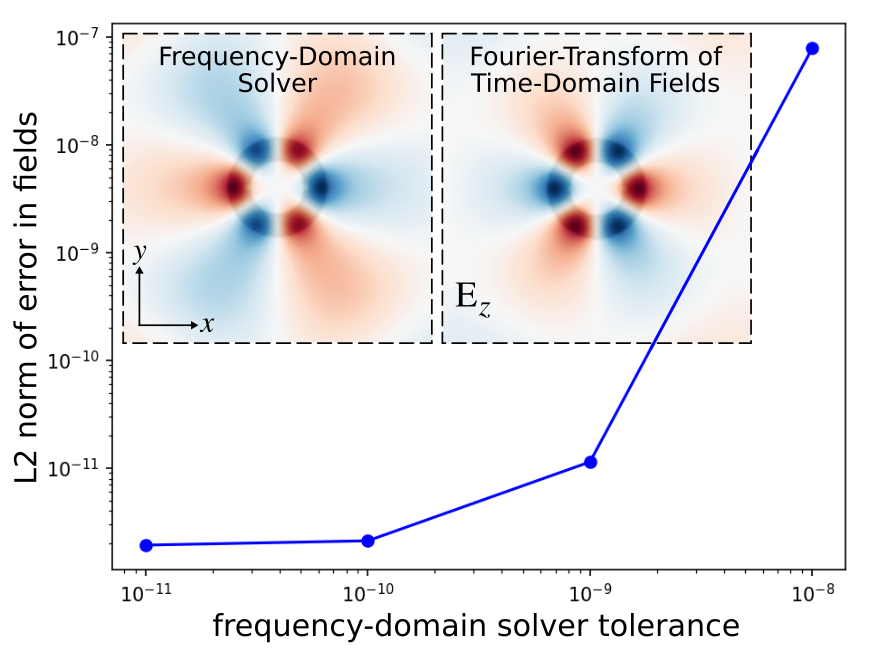# Frequency Domain Solver

This tutorial demonstrates Meep's frequency-domain solver which is used to compute the fields produced in response to a continuous-wave (CW) source. For a description of its inner workings, see Section 5.3 ("Frequency-domain solver") of Computer Physics Communications, Vol. 181, pp. 687-702, 2010. This example involves using the frequency-domain solver to compute the fields of a ring resonator which is described in Tutorial/Basics/Modes of a Ring Resonator. First, we will verify that the error in the fields decreases monotonically with decreasing tolerance of the iterative solver. And then, we will demonstrate qualitative agreement with the frequency-domain fields computed using a different method: Fourier transforming the time-domain fields in response to a narrowband Gaussian-pulse source.

Usage of the frequency-domain solver involves only two changes to the original simulation: (1) replace the pulse source with a continuous source, and (2) turn on complex fields since real fields are used by default. Everything else remains unchanged.

Since the frequency-domain solver uses an iterative method, there are a couple of things we can do to improve its convergence properties: (1) use a non-zero smoothing width for the CW source (default is 0) to reduce the high-frequency oscillations produced by its abrupt turn on which have slow group velocities and are absorbed poorly by PML, and (2) increase the $L$ parameter of the BiCGSTAB-L iterative solver from the default of 2 to 10.

We compute the fundamental mode at five different solver tolerances chosen on a logarithmic scale. We will then plot the L2 norm of the error in the fields as a function of the tolerance.

The simulation script is in examples/solve-cw.py. The notebook is examples/solve-cw.ipynb.

import meep as mp
import numpy as np
from numpy import linalg as LA
import matplotlib.pyplot as plt

n = 3.4
w = 1
r = 1
dpml = 2

cell_size = mp.Vector3(sxy,sxy)

pml_layers = [mp.PML(dpml)]

nonpml_vol = mp.Volume(mp.Vector3(), size=mp.Vector3(sxy-2*dpml,sxy-2*dpml))

fcen = 0.118

src = [mp.Source(mp.ContinuousSource(fcen),
component=mp.Ez,
center=mp.Vector3(r+0.1)),
mp.Source(mp.ContinuousSource(fcen),
component=mp.Ez,
center=mp.Vector3(-(r+0.1)),
amplitude=-1)]

symmetries = [mp.Mirror(mp.X,phase=-1),
mp.Mirror(mp.Y,phase=+1)]

sim = mp.Simulation(cell_size=cell_size,
geometry=geometry,
sources=src,
resolution=10,
force_complex_fields=True,
symmetries=symmetries,
boundary_layers=pml_layers)

num_tols = 5
tols = np.power(10, np.arange(-8.0,-8.0-num_tols,-1.0))
ez_dat = np.zeros((122,122,num_tols), dtype=np.complex_)

for i in range(num_tols):
sim.init_sim()
sim.solve_cw(tols[i], 10000, 10)
ez_dat[:,:,i] = sim.get_array(vol=nonpml_vol, component=mp.Ez)

err_dat = np.zeros(num_tols-1)
for i in range(num_tols-1):
err_dat[i] = LA.norm(ez_dat[:,:,i]-ez_dat[:,:,num_tols-1])

plt.figure(dpi=150)
plt.loglog(tols[:num_tols-1], err_dat, 'bo-');
plt.xlabel("frequency-domain solver tolerance");
plt.ylabel("L2 norm of error in fields");
plt.show()

eps_data = sim.get_array(vol=nonpml_vol, component=mp.Dielectric)
ez_data = np.real(ez_dat[:,:,num_tols-1])

plt.figure()
plt.imshow(eps_data.transpose(), interpolation='spline36', cmap='binary')
plt.imshow(ez_data.transpose(), interpolation='spline36', cmap='RdBu', alpha=0.9)
plt.axis('off')
plt.show()

if np.all(np.diff(err_dat) < 0):
print("PASSED solve_cw test: error in the fields is decreasing with increasing resolution")
else:
print("FAILED solve_cw test: error in the fields is NOT decreasing with increasing resolution")


The results are shown in the figure below. The error in the fields decreases monotonically with decreasing tolerance of the frequency-domain solver. The error is converging to an asymptotic limit of 1e-12 which is set by the lowest tolerance.As a further validation of the frequency-domain solver, we will compare its fields with those computed using time-stepping. This involves taking the Fourier transform of $E_z$ via the add_dft_fields routine. After the time stepping, the frequency-domain fields are accessed using get_dft_array.

sim.reset_meep()

src = [mp.Source(mp.GaussianSource(fcen,fwidth=df),
component=mp.Ez,
center=mp.Vector3(r+0.1)),
mp.Source(mp.GaussianSource(fcen,fwidth=df),
component=mp.Ez,
center=mp.Vector3(-(r+0.1)),
amplitude=-1)]

sim = mp.Simulation(cell_size=mp.Vector3(sxy,sxy),
geometry=geometry,
sources=src,
resolution=10,
symmetries=symmetries,
boundary_layers=pml_layers)

dft_obj = sim.add_dft_fields([mp.Ez], fcen, 0, 1, where=nonpml_vol)

sim.run(until_after_sources=100)

eps_data = sim.get_array(vol=nonpml_vol, component=mp.Dielectric)
ez_data = np.real(sim.get_dft_array(dft_obj, mp.Ez, 0))

plt.figure()
plt.imshow(eps_data.transpose(), interpolation='spline36', cmap='binary')
plt.imshow(ez_data.transpose(), interpolation='spline36', cmap='RdBu', alpha=0.9)
plt.axis('off')
plt.show()


The left inset of the figure above shows the real part of the scalar $E_z$ field, computed using the frequency-domain solver with a tolerance of 1e-12, superimposed on the ring-resonator geometry. Note the three-fold mirror symmetry of the field pattern (fundamental mode) and faint presence of the point source. The right inset is for the Fourier-transformed fields of the time-domain calculation. There is a phase difference in the field profiles which are otherwise qualitatively similar.